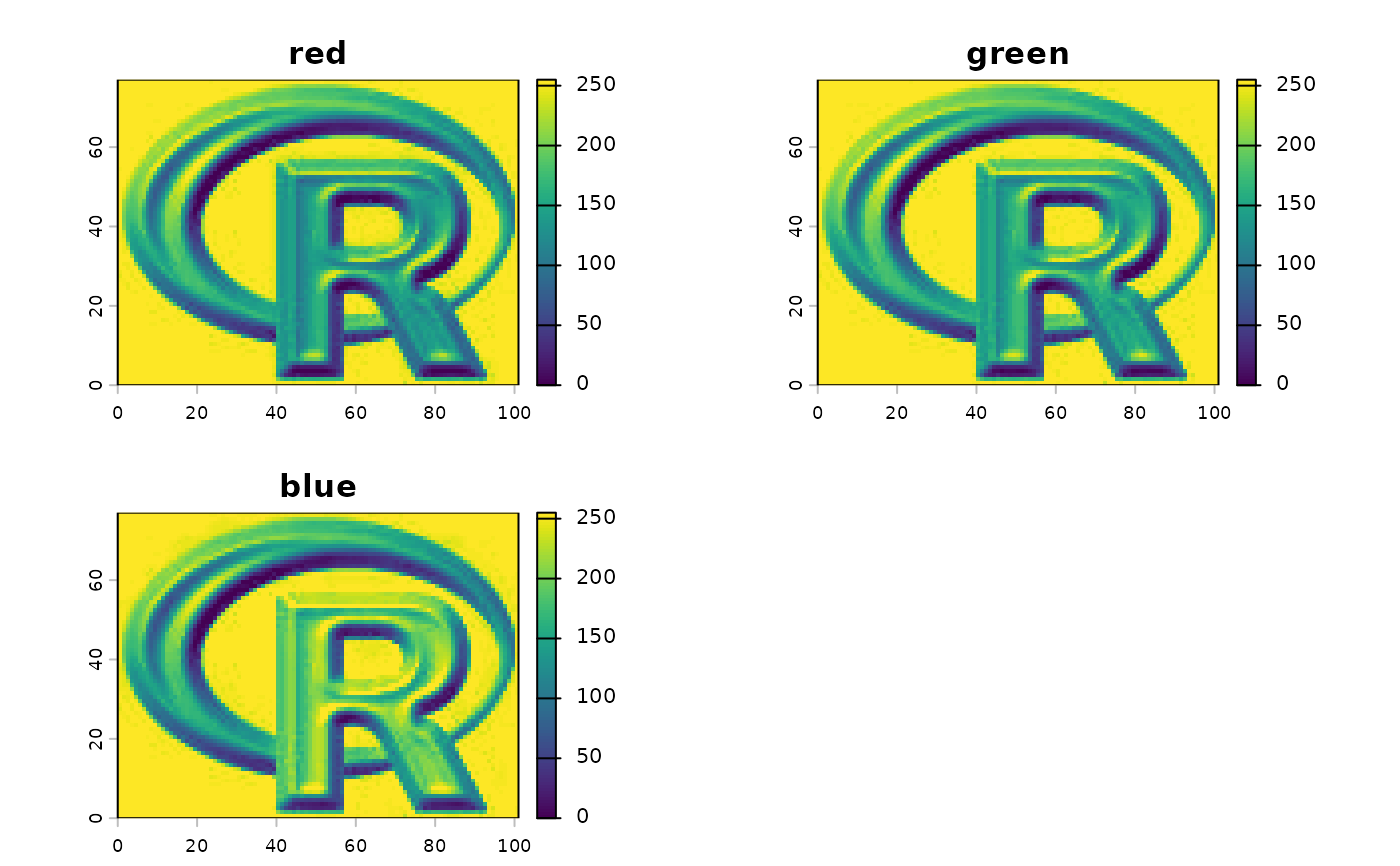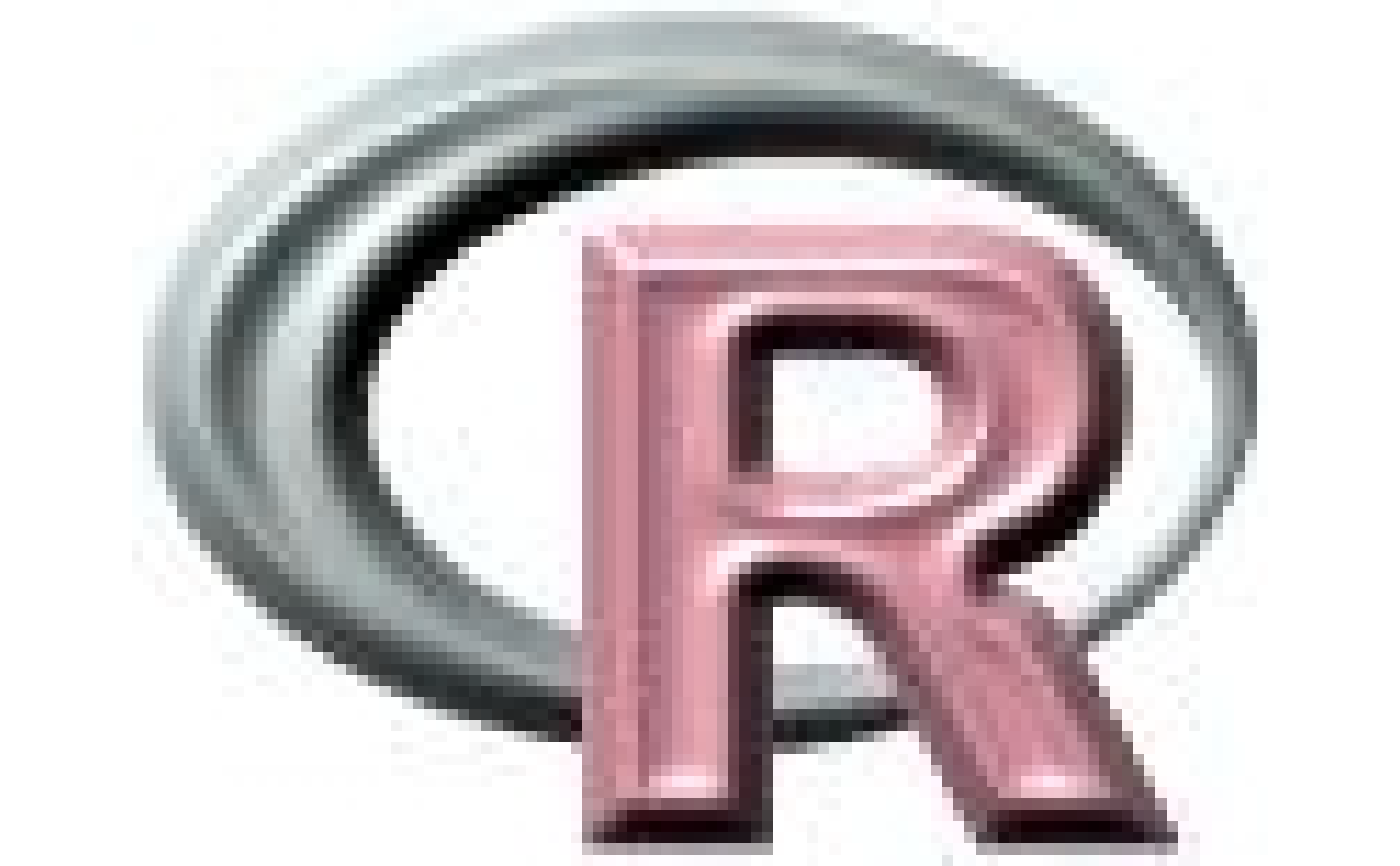With RGB you can get or set the layers to be used as Red, Green and Blue when plotting a SpatRaster. Currently, a benefit of this is that plot will send the object to plotRGB. You can also associated the layers with another color space (HSV, HSI or HSL)

With colorize you can convert a three-layer RGB SpatRaster into other color spaces. You can also convert it into a single-layer SpatRaster with a color-table.

# S4 method for SpatRaster
RGB(x)

# S4 method for SpatRaster
RGB(x, type="rgb")<-value

# S4 method for SpatRaster
colorize(x, to="hsv", alpha=FALSE, stretch=NULL,
grays=FALSE, NAzero=FALSE, filename="", overwrite=FALSE, ...)

# S4 method for SpatRaster
has.RGB(x, strict=TRUE)

## Arguments

x

SpatRaster

value

vector of three (or four) positive integers indicating the layers that are red, green and blue (and optionally a fourth transparency layer). Or NULL to remove the RGB settings

type

character. The color space. One of "rgb" "hsv", "hsi" and "hsl"

to

character. The color space to transform the values to. If x has RGB set, you can transform these to "hsv", "hsi" and "hsl", or use "col" to create a single layer with a color table. You can also use "rgb" to back transform to RGB

alpha

logical. Should an alpha (transparency) channel be included? Only used if x has a color-table and to="rgb"

stretch

character. Option to stretch the values to increase contrast: "lin" (linear) or "hist" (histogram). Only used for transforming RGB to col

grays

logical. If TRUE, a gray-scale color-table is created. Only used for transforming RGB to col

NAzero

logical. If TRUE, NAs are treated as zeros such that a color can be returned if at least one of the three channels has a value. Only used for transforming RGB to ("col")

strict

logical. If TRUE, the function returns FALSE if a color space such as "hsv", "hsi" and "hsl" is used

filename

character. Output filename

overwrite

logical. If TRUE, filename is overwritten

...

additional arguments for writing files as in writeRaster

## Examples

r <- rast(system.file("ex/logo.tif", package="terra"))
plot(r)has.RGB(r)
#>  TRUE
RGB(r) <- NULL
has.RGB(r)
#>  FALSE
plot(r)RGB(r) <- c(3,1,2)
plot(r)RGB(r) <- 1:3
x <- colorize(r, "col")
y <- colorize(r, "hsv")
z <- colorize(y, "rgb")